# HOMER Pro 3.11

 Navigation: Glossary Weibull DistributionThe two-parameter Weibull distribution is often used to characterize wind regimes because it has been found to provide a good fit with measured wind data. The probability density function is given by the following equation: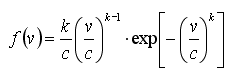where: v = the wind speed [m/s] k = the Weibull shape factor [unitless] c = the Weibull scale parameter [m/s]

The cumulative distribution function is given by the following equation: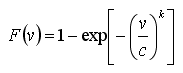The following equation relates the two Weibull parameters and the average wind speed: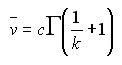where: Γ = the gamma function

One can describe a Weibull distribution using an average wind speed and a Weibull k value. The graph below shows five Weibull distributions, all with the same average wind speed of 6 m/s, but each with a different Weibull k value. As the graph shows, lower k values correspond to broader distributions.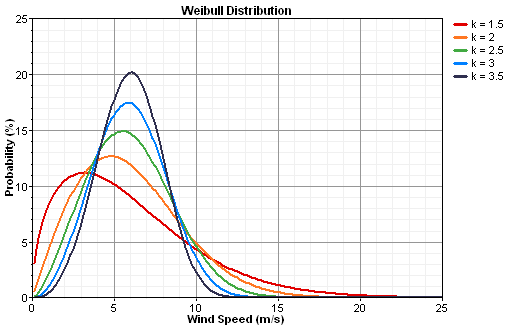To fit a Weibull distribution to measured wind data, HOMER uses the maximum likelihood method given by Stevens and Smulders, 1979. The graph below shows a typical distribution of wind speeds and the best-fit Weibull distribution.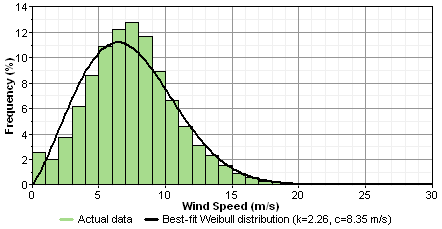See also

Weibull k Value